# 2021 Fantasy Football Player RankingsDeveloping accurate fantasy football player rankings is a key component of creating reliable fantasy football cheat sheets, and as we know your cheat sheet could make or break your fantasy football draft. In modern fantasy football leagues, almost any football position can be added to your fantasy league scoring configuration (even defensive players).

Regardless of your league’s configuration, you’ll need to be sure to have accurate NFL player rankings for each position that is required to complete your fantasy roster. Otherwise, how would you know how to make picks for your fantasy football draft boards as the season approachs? Here are some factors we consider when developing our fantasy football player rankings:

• Statistics- Obviously the stats from the previous season are most critical but as the season progresses current statistics become more relevant.
• Fantasy Point Output- Understand how each player’s statistics translate to point output based on your fantasy league configuration. Just as statistic/point-values vary from league to league, a player's ranking will likewise rise or fall based on league settings.
• Games Played- The number of games a player actually played in during the previous season is an oft-forgotten statistic. Would you rather draft a player that put up 80 fantasy points in 16 games, or a player who put up 60 fantasy points in 8 games? This is why FPPG output is a key statistic to follow (and also why it is integrated into all of our sheets by default).
• Team- The team a player plays for should definitely factor into their ranking, especially if the player has changed teams. Those teams with high-powered offenses will have players who put up more fantasy points, so remember to factor in the team when you configure your rankings.
• Experience- The factor that experience plays in your NFL player rankings is heavily dependent on the position being analyzed; a productive kicker will have a much longer career than a productive running back. Compare each player’s stats to their experience to ensure they are not on the downside of their career.
• Position on Depth Chart- As training camp battles progress, a clearer picture of each team’s depth chart should arise. Know which players have cemented a starting job and which players are still battling to move up the depth chart.
• Injury Concerns– Football players are constantly battling injuries, but some injuries are worse than others. Those players with injury concerns should slide down your player rankings; how far they slide will depend on the severity (and sometimes the frequency) of their injury.

There are many different factors to consider when configuring your 2021 NFL player rankings. The key to success is examining the relevant factors and properly applying them to the position and player being analyzed. We hope that the rankings provided will help you create reliable and accurate fantasy football cheat sheets in preparation for your 2021 fantasy football draft. Below are our top 5 players at each position named in the standard fantasy football scoring system. To view all rankings for a particular position please click the links above.

## Top 10 Quarterbacks

(view all)
1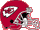Patrick Mahomes #13
 KC Chiefs, 3 years exp
GAM=15, PAYD=4740, PATD=38, RUCA=62, RUYD=308, RUTD=2, INT=6, FUM=2, FPPG=29.6, TFP=444
2Josh Allen #13
 BUF Bills, 3 years exp
GAM=16, PAYD=4544, PATD=37, RUCA=102, RUYD=421, RUTD=8, INT=10, FUM=6, FPPG=28.9, TFP=462
3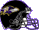Lamar Jackson #13
 BAL Ravens, 3 years exp
GAM=15, PAYD=2757, PATD=26, RUCA=159, RUYD=1005, RUTD=7, INT=9, FUM=4, FPPG=25.5, TFP=383
4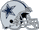Dak Prescott #12
 DAL Cowboys, 5 years exp
GAM=5, PAYD=1856, PATD=9, RUCA=18, RUYD=93, RUTD=3, INT=4, FUM=3, FPPG=28.3, TFP=142
5Russell Wilson #3
 SEA Seahawks, 9 years exp
GAM=16, PAYD=4212, PATD=40, RUCA=83, RUYD=513, RUTD=2, INT=13, FUM=4, FPPG=27.4, TFP=438
6Aaron Rodgers #12
 GB Packers, 16 years exp
GAM=16, PAYD=4299, PATD=48, RUCA=38, RUYD=149, RUTD=3, INT=5, FUM=2, FPPG=29.9, TFP=479
7Kyler Murray #13
 ARI Cardinals, 2 years exp
GAM=16, PAYD=3971, PATD=26, RUCA=133, RUYD=819, RUTD=11, INT=12, FUM=4, FPPG=26.9, TFP=431
8TB Buccaneers, 21 years exp
GAM=16, PAYD=4633, PATD=40, RUCA=30, RUYD=6, RUTD=3, INT=12, FUM=1, FPPG=26.1, TFP=418
9Justin Herbert #10
 LAC Chargers, 1 years exp
GAM=15, PAYD=4336, PATD=31, RUCA=55, RUYD=234, RUTD=5, INT=10, FUM=1, FPPG=26.1, TFP=391
10Joe Burrow #13
 CIN Bengals, 1 years exp
GAM=10, PAYD=2688, PATD=13, RUCA=37, RUYD=142, RUTD=3, INT=5, FUM=4, FPPG=20, TFP=200

## Top 10 Running Backs

(view all)
1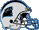Christian McCaffrey #34
 CAR Panthers, 4 years exp
GAM=3, RUCA=59, RUYD=225, RUTD=5, RETR=19, RECP=17, REYD=149, RETD=1, FUM=0, FPPG=24.5, TFP=73
2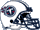Derrick Henry #34
 TEN Titans, 5 years exp
GAM=16, RUCA=378, RUYD=2027, RUTD=17, RETR=31, RECP=19, REYD=114, RETD=0, FUM=2, FPPG=19.5, TFP=312
3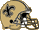Alvin Kamara #34
 NO Saints, 4 years exp
GAM=15, RUCA=187, RUYD=932, RUTD=16, RETR=107, RECP=83, REYD=756, RETD=5, FUM=0, FPPG=19.7, TFP=295
4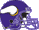Dalvin Cook #34
 MIN Vikings, 4 years exp
GAM=14, RUCA=312, RUYD=1557, RUTD=16, RETR=54, RECP=44, REYD=361, RETD=1, FUM=3, FPPG=20.6, TFP=288
5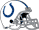Jonathan Taylor #34
 IND Colts, 1 years exp
GAM=15, RUCA=232, RUYD=1169, RUTD=11, RETR=39, RECP=36, REYD=299, RETD=1, FUM=1, FPPG=14.5, TFP=217
6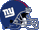Saquon Barkley #0
 NYG Giants, 3 years exp
GAM=2, RUCA=19, RUYD=34, RUTD=0, RETR=9, RECP=6, REYD=60, RETD=0, FUM=0, FPPG=4.7, TFP=9
7Aaron Jones #34
 GB Packers, 4 years exp
GAM=14, RUCA=201, RUYD=1104, RUTD=9, RETR=63, RECP=47, REYD=355, RETD=2, FUM=0, FPPG=15.1, TFP=212
8Nick Chubb #34
 CLE Browns, 3 years exp
GAM=12, RUCA=190, RUYD=1067, RUTD=12, RETR=18, RECP=16, REYD=150, RETD=0, FUM=1, FPPG=16, TFP=192
9Austin Ekeler #30
 LAC Chargers, 4 years exp
GAM=10, RUCA=116, RUYD=530, RUTD=1, RETR=65, RECP=54, REYD=403, RETD=2, FUM=0, FPPG=11.1, TFP=111
10Ezekiel Elliott #34
 DAL Cowboys, 5 years exp
GAM=15, RUCA=244, RUYD=979, RUTD=6, RETR=71, RECP=52, REYD=338, RETD=2, FUM=5, FPPG=11.3, TFP=170

(view all)
1GB Packers, 7 years exp
GAM=14, RETR=149, RECP=115, REYD=1374, RETD=18, RUYD=0, RUTD=0, FUM=1, FPPG=17.4, TFP=243
2Tyreek Hill #80
 KC Chiefs, 5 years exp
GAM=15, RETR=135, RECP=87, REYD=1276, RETD=15, RUYD=123, RUTD=2, FUM=0, FPPG=16.1, TFP=242
3Stefon Diggs #14
 BUF Bills, 6 years exp
GAM=16, RETR=166, RECP=127, REYD=1535, RETD=8, RUYD=1, RUTD=0, FUM=0, FPPG=12.6, TFP=202
4DeAndre Hopkins #10
 ARI Cardinals, 8 years exp
GAM=16, RETR=160, RECP=115, REYD=1407, RETD=6, RUYD=1, RUTD=0, FUM=2, FPPG=10.8, TFP=173
5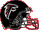Calvin Ridley #18
 ATL Falcons, 3 years exp
GAM=15, RETR=143, RECP=90, REYD=1374, RETD=9, RUYD=1, RUTD=0, FUM=1, FPPG=12.6, TFP=190
6Michael Thomas #80
 NO Saints, 5 years exp
GAM=7, RETR=55, RECP=40, REYD=438, RETD=0, RUYD=1, RUTD=0, FUM=0, FPPG=6.3, TFP=44
7A.J. Brown #80
 TEN Titans, 2 years exp
GAM=14, RETR=106, RECP=70, REYD=1075, RETD=11, RUYD=0, RUTD=0, FUM=1, FPPG=12.2, TFP=172
8D.K. Metcalf #80
 SEA Seahawks, 1 years exp
GAM=16, RETR=129, RECP=83, REYD=1303, RETD=10, RUYD=0, RUTD=0, FUM=1, FPPG=11.8, TFP=188
9Justin Jefferson #80
 MIN Vikings, 1 years exp
GAM=16, RETR=125, RECP=88, REYD=1400, RETD=7, RUYD=2, RUTD=0, FUM=0, FPPG=11.4, TFP=182
10Terry McLaurin #80
 WAS Team, 2 years exp
GAM=15, RETR=134, RECP=87, REYD=1118, RETD=4, RUYD=30, RUTD=0, FUM=1, FPPG=9.1, TFP=137

## Top 10 Tight Ends

(view all)
1Travis Kelce #87
 KC Chiefs, 8 years exp
GAM=15, RETR=145, RECP=105, REYD=1416, RETD=11, RUYD=0, RUTD=0, FUM=1, FPPG=13.7, TFP=206
2Darren Waller #85
 LV Raiders, 2 years exp
3George Kittle #85
 SF 49ers, 4 years exp
GAM=8, RETR=63, RECP=48, REYD=634, RETD=2, RUYD=17, RUTD=0, FUM=0, FPPG=9.6, TFP=77
4Mark Andrews #89
 BAL Ravens, 3 years exp
GAM=14, RETR=88, RECP=58, REYD=701, RETD=7, RUYD=0, RUTD=0, FUM=0, FPPG=8, TFP=112
5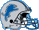T.J. Hockenson #88
 DET Lions, 2 years exp
GAM=16, RETR=101, RECP=67, REYD=723, RETD=6, RUYD=0, RUTD=0, FUM=1, FPPG=6.6, TFP=106
6Kyle Pitts #84
 ATL Falcons, Rookie
7Dallas Goedert #85
 PHI Eagles, 3 years exp
GAM=11, RETR=65, RECP=46, REYD=524, RETD=3, RUYD=0, RUTD=0, FUM=0, FPPG=6.4, TFP=70
8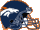Noah Fant #88
 DEN Broncos, 2 years exp
GAM=14, RETR=93, RECP=62, REYD=673, RETD=3, RUYD=0, RUTD=0, FUM=0, FPPG=6.1, TFP=85
9Logan Thomas #5
 WAS Team, 7 years exp
GAM=16, RETR=110, RECP=72, REYD=670, RETD=6, RUYD=5, RUTD=0, FUM=0, FPPG=6.5, TFP=104
10Irv Smith #85
 MIN Vikings, 1 years exp
GAM=12, RETR=43, RECP=30, REYD=365, RETD=5, RUYD=0, RUTD=0, FUM=0, FPPG=5.5, TFP=66

## Top 10 Kickers

(view all)
1Justin Tucker #9
 BAL Ravens, 9 years exp
GAM=16, MAFG=26, MIFG=3, MAXP=52, MIXP=1, FPPG=7.5, TFP=120
2Younghoe Koo #7
 ATL Falcons, 4 years exp
GAM=15, MAFG=37, MIFG=2, MAXP=33, MIXP=3, FPPG=9, TFP=135
3Harrison Butker #8
 KC Chiefs, 4 years exp
GAM=16, MAFG=25, MIFG=2, MAXP=48, MIXP=6, FPPG=6.9, TFP=111
4Will Lutz #3
 NO Saints, 5 years exp
GAM=16, MAFG=23, MIFG=5, MAXP=57, MIXP=1, FPPG=6.9, TFP=110
5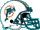Jason Sanders #3
 MIA Dolphins, 3 years exp
GAM=16, MAFG=36, MIFG=3, MAXP=36, MIXP=0, FPPG=8.4, TFP=135
6Rodrigo Blankenship #3
 IND Colts, 1 years exp
GAM=16, MAFG=32, MIFG=5, MAXP=43, MIXP=2, FPPG=7.6, TFP=122
7Tyler Bass #2
 BUF Bills, 1 years exp
GAM=16, MAFG=28, MIFG=6, MAXP=57, MIXP=2, FPPG=7.6, TFP=121
8Jason Myers #2
 SEA Seahawks, 6 years exp
GAM=16, MAFG=24, MIFG=0, MAXP=49, MIXP=4, FPPG=7.3, TFP=117
9Greg Zuerlein #4
 DAL Cowboys, 9 years exp
GAM=16, MAFG=34, MIFG=7, MAXP=33, MIXP=3, FPPG=6.9, TFP=111
10Robbie Gould #9
 SF 49ers, 16 years exp
GAM=15, MAFG=19, MIFG=4, MAXP=36, MIXP=2, FPPG=5.3, TFP=79

## Top 10 Defenses/Special Teams

(view all)
1Los Angeles Rams
GAM=16, FREC=8, INT=14, SACK=53, DTD=4, PA=296, FPPG=7.8, TFP=125
2San Francisco 49ers
GAM=16, FREC=8, INT=12, SACK=30, DTD=1, PA=390, FPPG=4.8, TFP=76
3Washington Redskins
GAM=16, FREC=7, INT=16, SACK=47, DTD=3, PA=329, FPPG=7.2, TFP=115
4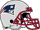New England Patriots
GAM=16, FREC=4, INT=18, SACK=24, DTD=2, PA=353, FPPG=5, TFP=80
5Baltimore Ravens
GAM=16, FREC=12, INT=10, SACK=39, DTD=3, PA=303, FPPG=6.8, TFP=109
6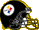Pittsburgh Steelers
GAM=16, FREC=9, INT=18, SACK=56, DTD=3, PA=312, FPPG=8.2, TFP=132
7Denver Broncos
GAM=16, FREC=6, INT=10, SACK=42, DTD=0, PA=446, FPPG=4.6, TFP=74
8Cleveland Browns
GAM=16, FREC=10, INT=11, SACK=38, DTD=2, PA=419, FPPG=6.2, TFP=100
9Indianapolis Colts
GAM=16, FREC=10, INT=15, SACK=40, DTD=4, PA=362, FPPG=7.9, TFP=126
10Buffalo Bills
GAM=16, FREC=11, INT=15, SACK=38, DTD=3, PA=375, FPPG=6.8, TFP=108